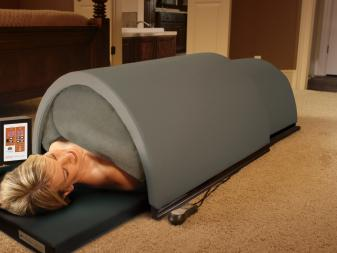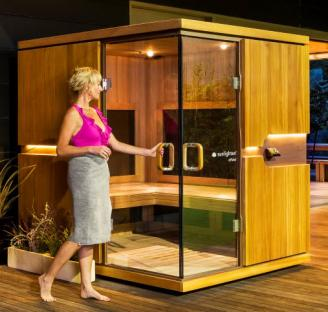August 09, 2022

# Running costs of an infrared sauna

Light Science

When looking at a sauna for home or business, people often wonder about the running costs.

The good news is infrared saunas run on 240V power and offer much lower electricity and maintenance costs than traditional saunas.

So to help you, we have put together a summary of the approximate electricity costs of each of our saunas.

Please note that these are our examples only.

To calculate how much electricity an electrical appliance uses

First, find the power rating of the appliance. This can be displayed on a label with other notes like the model number or serial number.

Often the power rating of an appliance is shown in watts (W). So we need to convert this to kilowatts (KW) by dividing the number of watts (W) by 1000. Once we have the power rating in KW, this shows the KW units of electricity the appliance will use each hour (KWh).

Example only:The Solo Sauna

• (domes and SoloPad) uses 1260 W divided by 1000 = 1.26 KWh (units of electricity each hour).
• At peak time the cost of electricity per kilowatt hour was approx. 34 cents per KWh.
• So the cost of running the Solo System during peak time was 34 cents x 1.26 = 42.8 cents per hour.
• At off peak time the cost of electricity per kilowatt hour was approx. 17 cents per KWh.
• So the cost of running the Solo System during off peak time was 17 cents x 1.26 = 21.4 cents per hourThe Signature Range

The Signature 1 person

• Uses 1673 W divided by 1000 = 1.67 KWh (units of electricity each hour).
• At peak time the cost of electricity per kilowatt hour was approx. 34 cents per KWh.
• The cost of running the Signature 1 during peak time was 34 cents x 1.67 = 56.8 cents per hour.
• At off peak time the cost of electricity per kilowatt hour was approx. 17 cents per KWh.
• The cost of running the Signature 1 during off peak time was 17 cents x 1.67 = 28.4 cents per hour

The Signature 2 person

• Uses 2251 W divided by 1000 = 2.25 KWh (units of electricity each hour).
• At peak time the cost of electricity per kilowatt hour was approx. 34 cents per KWh.
• The cost of running the Signature 2 during peak time was 34 cents x 2.25 = 76.5 cents per hour.
• At off peak time the cost of electricity per kilowatt hour was approx. 17 cents per KWh.
• The cost of running the Signature 2 during off peak time was 17 cents x 2.25 = 38.3 cents per hour

The Signature 3 person

• Uses 2551 W divided by 1000 = 2.55 KWh (units of electricity each hour).
• At peak time the cost of electricity per kilowatt hour was approx. 34 cents per KWh.
• The cost of running the Signature 3 during peak time was 34 cents x 2.03 = 86.7 cents per hour.
• At off peak time the cost of electricity per kilowatt hour was approx. 17 cents per KWh.
• The cost of running the Signature 3 during off peak time was 17 cents x 2.55 = 43.4 cents per hour

The Signature 4 person

• Uses 3031 W divided by 1000 = 3.03 KWh (units of electricity each hour).
• At peak time the cost of electricity per kilowatt hour was approx. 34 cents per KWh.
• The cost of running the Signature 4 during peak time was 34 cents x 2.50 = 1.03 cents per hour.
• At off peak time the cost of electricity per kilowatt hour was approx. 17 cents per KWh.
• The cost of running the Signature 4 during off peak time was 17 cents x 3.03 = 51.5 cents per hourThe mPulse Range

The mPulse 1 person ‘Aspire’

• Uses 1800 W divided by 1000 = 1.8 KWh (units of electricity each hour).
• At peak time the cost of electricity per kilowatt hour was approx. 34 cents per KWh.
• The cost of running the mPulse ‘Aspire’ during peak time was 34 cents x 1.8 = 61.2 cents per hour.
• At off peak time the cost of electricity per kilowatt hour was approx. 17 cents per KWh.
• The cost of running the mPulse ‘Aspire’ during off peak time was 17 cents x 1.8 = 30.6 cents per hour

The mPulse 2 person ‘Believe’

• Uses 2400 W divided by 1000 = 2.4 KWh (units of electricity each hour).
• At peak time the cost of electricity per kilowatt hour was approx. 34 cents per KWh.
• The cost of running the mPulse ‘Believe’ during peak time was 34 cents x 2.4 = 81.6 cents per hour.
• At off peak time the cost of electricity per kilowatt hour was approx. 17 cents per KWh.
• The cost of running the mPulse ‘Believe’ during off peak time was 17 cents x 2.4 = 40.8 cents per hour

The mPulse 3 person ‘Conquer’

• Uses 3300 W divided by 1000 = 3.3 KWh (units of electricity each hour).
• At peak time the cost of electricity per kilowatt hour was approx. 34 cents per KWh.
• The cost of running the mPulse ‘Conquer’ during peak time was 34 cents x 3.3 = \$1.12 per hour.
• At off peak time the cost of electricity per kilowatt hour was approx. 17 cents per KWh.
• The cost of running the mPulse ‘Conquer’ during off peak time was 17 cents x 3.3 = 56 cents per hour

The mPulse 4 person ‘Discover’

• Uses 4200 W divided by 1000 = 4.2 KWh (units of electricity each hour).
• At peak time the cost of electricity per kilowatt hour was approx. 34 cents per KWh.
• The cost of running the mPulse ‘Discover’ during peak time was 34 cents x 4.2 = \$1.42 per hour.
• At off peak time the cost of electricity per kilowatt hour was approx. 17 cents per KWh.
• The cost of running the mPulse ‘Discover’ during off peak time was 17 cents x 4.2 = 71.4 cents per hour

The mPulse 5-6 person ‘Empower’

• Uses 4800 W divided by 1000 = 4.8 KWh (units of electricity each hour).
• At peak time the cost of electricity per kilowatt hour was approx. 34 cents per KWh.
• The cost of running the mPulse ‘Empower’ during peak time was 34 cents x 4.8 = \$1.63 per hour.
• At off peak time the cost of electricity per kilowatt hour was approx. 17 cents per KWh.
• The cost of running the mPulse ‘Empower’ during off peak time was 17 cents x 4.8 = 81.6 cents per hour

Your cost per KWh may be different

For the exact cost of running your appliance, find out what your electricity supplier charges per kilowatt hour. This is noted on your electricity bill in cents per unit (KWh).